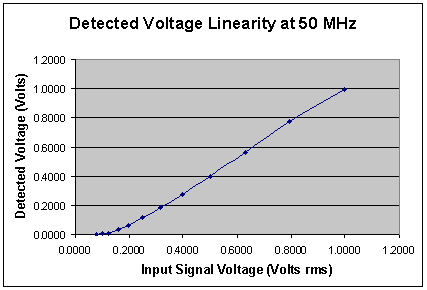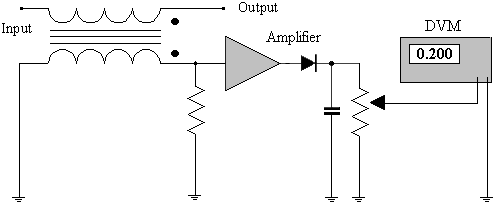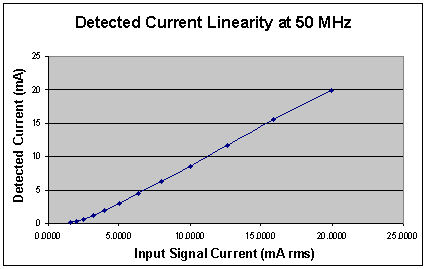# Appendix C - Voltage / Current Detector page 2

The voltage readings are taken at each power level in the spreadsheet and the voltage indicated by the DMM entered into the third column (C). From the second and third columns (B&C), an xy chart can be constructed that is similar to Figure 1.3.

Figure 1.3It can be seen in Figure 1.3 that the linearity of the diode pump circuit is poor below an input level of about 200 mV rms. The curve has now become a near square law and this can be used to advantage if a low power measurement is being made. However, we are measuring voltage and in order to make the voltage detection more linear using calculation, we first find the constants for the formula that fit the curve. This can be performed in Excel using the LINEST Function. Simply select the function and enter the DMM reading and the input voltage as the x and y data respectively. Excel will calculate results and these are required in the form y = b + m1x + m2x^2 + m3x^3 + m4x^4 which translates into Actual Voltage = b + m1*(DMM Reading)+ m2*(DMM Reading)^2 + m3*(DMM Reading)^3 + m4*(DMM Reading)^4. Whenever a reading is taken from the voltage detector output, just enter the DMM voltage reading into this formula with the appropriate constants and the correct signal voltage level will be calculated.

The input signal to the circuit is passed through a current transformer as can be seen in Figure 1.4. The resultant current in the secondary of the transformer develops a voltage across a load resistor to ground. This resistor flattens the frequency response and matches the transformer output impedance to the amplifier input impedance. The amplifier produces an output voltage level similar to the voltage level present at the signal input to the current transformer. This ensures that the detection diode on the output of the amplifier has adequate voltage to perform mainly in the linear part of the response curve and works in a similar voltage region to the voltage detection circuit described previously.

Figure 1.4The Voltage and Current Detector is connected directly to the output of the Signal Generator and is terminated with a 50 Ohm load as shown in Figure C.2. The Signal Generator output is set to +13 dBm (or 1 Volt rms) at a frequency of 50 MHz. The DMM is connected to the Voltage and Current Detector, current output and should indicate approximately 200 mV. This represents ten times the rms current of 20 mA in the primary of the current transformer ( If the output potentiometer of the detector circuit is adjusted to give a 20 mV reading it will be difficult to get a good reading at low input power levels when the detected dc voltage approaches the noise level of the DMM test leads).

The input current is calculated from simple Ohms law using the input voltage calculated in the second column (B) of Spreadsheet_01.

I = V / R

Change into mA and impedance

I = (V x 1000) / Z  Formula 1.3

For an input of +13 dBm or 0.9988 Volts this is:-

I = (0.9988 x 1000) / 50 = 19.98 mA

We now use Formula 1.3 to calculate the input current for the range of signal generator output voltages in the second column (B) in the spreadsheet. The calculated input current is placed in the fourth column (D). Current readings are taken at each power level in the spreadsheet and entered into the fifth column (E). From the fourth and fifth columns (D&E), a xy chart is generated that should appear similar to Figure 1.5.

Figure 1.5We now find the formula that fits the current curve. This can again be performed in Excel using the LINEST function. Simply select the function and enter the DMM reading divided by ten, and the input current as the x and y data respectively. Excel will calculate the constants for the formula in the form y = b + m1x + m2x^2 + m3x^3 + m4x^4, which now translates into actual current = b + m1x(DMM reading/10) + m2x(DMM reading/10)^2 + m3x(DMM reading/10)^3 + m4x(DMM reading/10)^4. Whenever a reading is taken from the current detector output, simply feed the result into this formula with the appropriate constants and the correct current is calculated.

In order to be able to interpret the results of measurements made on transmission lines, it is important to understand the basic functionality of the Voltage / Current Detector. In the voltage detector, the measured voltage is taken from the wiper of the potentiometer as shown in figure 1.1 and this is a total of the sampled INCIDENT and REFLECTED signals. As the sampled signals have very small currents of individual phases that pass in the same direction through the detector diode and  potentiometer to ground, the resulting potential at the wiper is a Vector Sum of the sampled INCIDENT and REFLECTED signals.

The measured current is sampled using a current transformer as shown in figure 1.4. In the primary of the current transformer the INCIDENT and REFLECTED currents flow in opposite directions and have individual phases. This causes the resultant current in the transformer secondary to be a Vector Difference of the two primary currents. This resultant current develops a voltage across the resistor which then gives the total input as a voltage to the amplifier and detector stage.

The V/C Detector finds the peak voltage and peak current of the signals present because the capacitors in the diode pump circuits retain the peak voltage from the detector diodes.

From this, it can be seen that the voltage detector gives an output of Peak Vector Sum Voltage and the current detector gives a voltage proportional to the Peak Vector Difference Current. It is important to keep this difference in mind when analysing results otherwise it is easy to wrongly assume that voltage and current are out of phase when they are in fact, in phase. This simple misinterpretation of the phase of the current and voltage measurements has caused many misconceptions in the past and the whole point of this measuring device and the accompanying study of transmission lines is to eliminate these misconceptions and understand the realities.

1    2      Page Number

Transmission Line Index

Technical Article Index